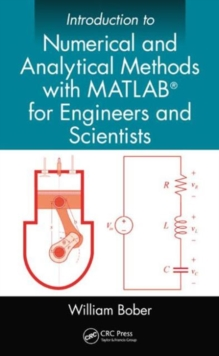Supporting your high street Find out how »
• My Account# Introduction to Numerical and Analytical Methods with MATLAB (R) for Engineers and Scientists Hardback

#### Description

Introduction to Numerical and Analytical Methods with MATLAB (R) for Engineers and Scientists provides the basic concepts of programming in MATLAB for engineering applications. * Teaches engineering students how to write computer programs on the MATLAB platform* Examines the selection and use of numerical and analytical methods through examples and case studies* Demonstrates mathematical concepts that can be used to help solve engineering problems, including matrices, roots of equations, integration, ordinary differential equations, curve fitting, algebraic linear equations, and moreThe text covers useful numerical methods, including interpolation, Simpson's rule on integration, the Gauss elimination method for solving systems of linear algebraic equations, the Runge-Kutta method for solving ordinary differential equations, and the search method in combination with the bisection method for obtaining the roots of transcendental and polynomial equations.

It also highlights MATLAB's built-in functions. These include interp1 function, the quad and dblquad functions, the inv function, the ode45 function, the fzero function, and many others.

The second half of the text covers more advanced topics, including the iteration method for solving pipe flow problems, the Hardy-Cross method for solving flow rates in a pipe network, separation of variables for solving partial differential equations, and the use of Laplace transforms to solve both ordinary and partial differential equations.

This book serves as a textbook for a first course in numerical methods using MATLAB to solve problems in mechanical, civil, aeronautical, and electrical engineering.

It can also be used as a textbook or as a reference book in higher level courses.

#### Information

• Format: Hardback
• Pages: 556 pages, Approx. 1,269 equations; 37 Tables, black and white; 208 Illustrations, black and white
• Publisher: Taylor & Francis Inc
• Publication Date:
• Category: Mathematics
• ISBN: 9781466576025

£105.00

£97.15

on all orders

###### Pick up orders

from local bookshops